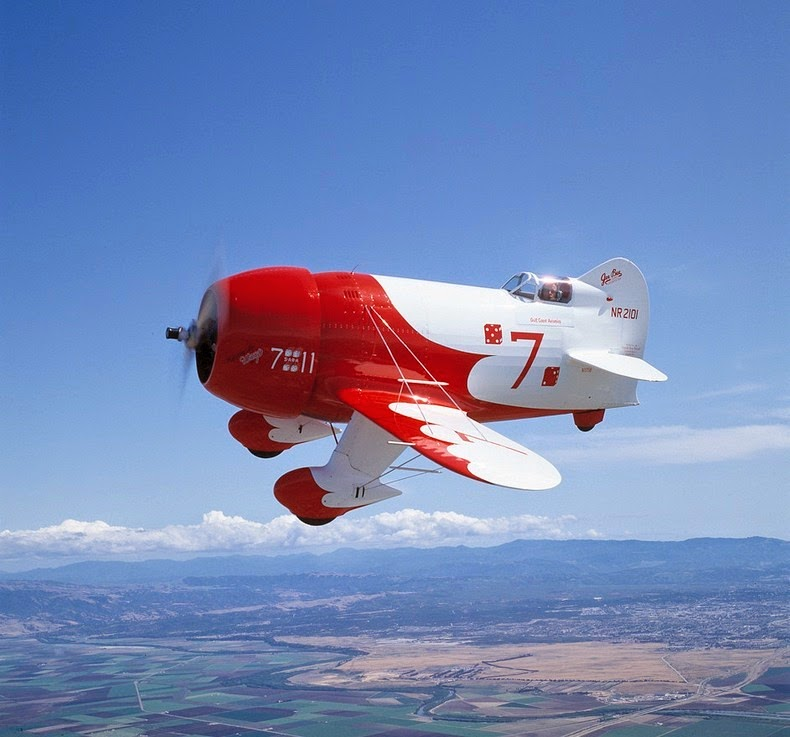# Flying highAn airplane, when in flight, is subjected to drag that is proportional to its speed $v$. But air flowing over the wings also pushes it downward. Given this consideration, we can model the total air resistance force on a plane as $F_f = av^2 + bv^{-2}$ for some constants $a$ and $b$ which depend on the airplane.

Consider a plane that is in a steady flight at which the engine must provide an equal and opposite force to the resistance force. The speed at which the airplane flies the longest distance given a specific amount of fuel can be expressed as $v = \left(K \times \frac{b}{a}\right)^\frac{1}{M}$ where $K$ and $M$ are positive integers. What is the value of $K + M$ ?

×